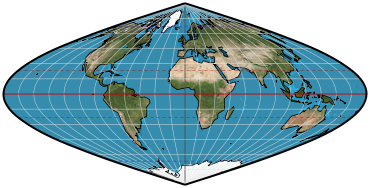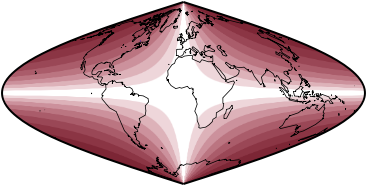## Geocart Projections

What is a projection?

Previous | Next

equal-area polynomialParameters: Note, Coefficient a, Coefficient b, Coefficient c, Coefficient d, Coefficient e

Classifications

Pseudocylindric
Equal-area

Graticule

Meridians: Complex curves equally spaced along parallels
Parallels: Straight parallel lines
Poles: Points
Symmetry: Symmetric east-west. Symmetric north-south when b and d = 0.

Parameters

Coefficients <i>a</i>, <i>b</i>, <i>c</i>, <i>d</i>, <i>e</i>, each for a successively higher power of the latitude. Generally these values should be 1.0 or less, and the higher the letter, the smaller the value.

Limiting forms

Sinsoidal, when a = 1.0 and the others = 0.

Origin

Developed by Daniel “daan” Strebe (1962–) in 2015. However, the concept is straightforward, with several polynomial developments appearing in the literature, typically for optimizing the projection in some way.